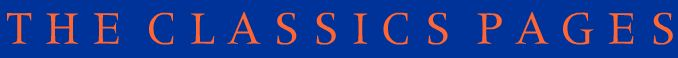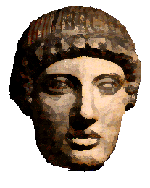# The Maths-Classics project

## Assignment 4

### Latin

We have seen that most of the mathematical terms are a combination of a prefix and a suffix. This section contains some from a Latin derivation.

Find out the Latin derivation ( i.e. Give the Latin word and its meaning) of the following prefixes and suffixes:

semi-

rect-

multi-

equi-

sec- ( sect- )

tang-

circ-

-angle

-lateral

Draw and clearly label the following geometrical terms; try to give a literal definition (from the Latin) and show how this has been used in the mathematical meaning.

rectangle

equilateral triangle

circle

secant

sector

segment

## CirclesWhat did arc originally mean? (use dictionary). Compare this with the French " arc en ciel " (what is this?)

What is a chord and why is it an appropriate name? (use dictionary to find out the derivation)

Draw a diagram to illustrate a circle with an arc and chord clearly shown.

Tangent: What are the literal and Mathematical meanings? Do you know a similar word for a South American dance? (why is it called this?). Draw a tangent on your circle diagram.